# Reason + multiplication principle - math problems

#### Number of problems found: 89

• Training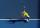The table contains tennis training schedule for Saturday's younger students during the winter indoor season. Before the start of the summer season is preparing a new training schedule. Tomas Kucera will be able to practice only in the morning, sisters Kov
• Logik game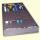Letter game Logik is a two player game, which has the following rules: 1. The first player thinks five-letter word in which no letter is not repeated. 2. The second player writes a five-letter word. 3. The first player answers two numbers - the first numb
• Pairs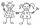From the five girls and four boys teachers have to choose one pair of boy and girl. A) How many such pairs of (M + F)? B) How many pairs where only boys (M + M)? C) How many are all possible pairs?
• Combi-triangle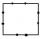On each side of the square is marked 10 different points outside the vertices of the square. How many triangles can be constructed from this set of points, where each vertex of the triangle lie on the other side of the square?
• Cinema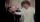How many ways can be divided 11 free tickets to the premiere of "Jáchyme throw it in the machine" between 6 pensioners?
• Toys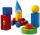3 children pulled 12 different toys from a box. Many ways can be divided toys so that each child had at least one toy?
• Hockey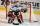The hockey match ended 8:2. How many different matches could be?
• Seating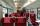How many ways can 6 people sit on 6 numbered chairs (e. g. , seat reservation on the train)?
• Phone numbersHow many 7-digit telephone numbers can be compiled from the digits 0,1,2,..,8,9 that no digit is repeated?

We apologize, but in this category are not a lot of examples.
Do you have an exciting math question or word problem that you can't solve? Ask a question or post a math problem, and we can try to solve it.

We will send a solution to your e-mail address. Solved examples are also published here. Please enter the e-mail correctly and check whether you don't have a full mailbox.

Reason - math problems. Multiplication principle - math problems.